## C++ Program to Generate Multiplication Table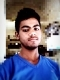Published on 27-Sep-2018 12:25:35
The multiplication table is used to define a multiplication operation for any number. It is normally used to lay the foundation of elementary arithmetic operations with base ten numbers.The multiplication table of any number is written till 10. In each row, the product of the number with 1 to 10 ... Read More

## C++ Program to Find Factorial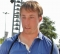Published on 27-Sep-2018 11:57:40
Factorial of a non-negative integer n is the product of all the positive integers that are less than or equal to n.For example: The factorial of 5 is 120.5! = 5 * 4 * 3 * 2 *1 5! = 120The factorial of an integer can be found using a ... Read More

## C++ Program to Check Leap YearPublished on 27-Sep-2018 11:52:45
A leap year contains one additional day that is added to keep the calendar year synchronized with the astronomical year.A year that is divisible by 4 is known as a leap year. However, years divisible by 100 are not leap years while those divisible by 400 are.The program that checks ... Read More

## Python program to count the elements in a list until an element is a Tuple?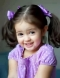Published on 26-Sep-2018 18:07:57
A is a given list. This list has nested tuples. Our task is to count the elements in a list until an element is a tuple. Here we use isinstance() function. This function has two parameters object and classinfo.object is to be checked and classinfo is class, type or tuple ... Read More

## C++ Program to Calculate Sum of Natural NumbersPublished on 26-Sep-2018 17:47:42
The natural numbers are the positive integers starting from 1.The sequence of natural numbers is −1, 2, 3, 4, 5, 6, 7, 8, 9, 10……Sum of the first n natural numbers can be calculated using the for loop or the formula.Programs specifying both of these methods are given as follows ... Read More

## Python program to count occurrences of an element in a tuple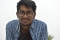Published on 26-Sep-2018 17:38:33
Here given a user input tuple, our task is to count occurrences of a given element in a tuple. Example Input : A = [10, 20, 30, 40, 10, 100, 80, 10] X = 10 Output : 3 Algorithm ... Read More

## C++ Program to Find All Roots of a Quadratic EquationPublished on 26-Sep-2018 17:38:59
A quadratic equation is in the form ax2 + bx + c. The roots of the quadratic equation are given by the following formula −There are three cases −b2 < 4*a*c - The roots are not real i.e. they are complexb2 = 4*a*c - The roots are real and both ... Read More

## C++ Program to Find Largest Number Among Three NumbersPublished on 26-Sep-2018 17:31:13
The largest number among three numbers can be found using if statement multiple times. This is given in a program as follows −Example Live Demo#include <iostream> using namespace std; int main() {    int a = 5 ,b = 1 ,c = 9;    if(a>b) {       if(a>c)   ... Read More

## Python program to count the number of vowels using set in a given string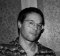Published on 26-Sep-2018 17:20:27
In this program, given a user input string. We have to count number of vowels in this string. Here we use set in Python. Set is an unordered collection data type that is iterable, mutable and no duplicate elements. Example Input : str1=pythonprogram Output : 3 Algorithm ... Read More

## C++ Program to Check Whether a character is Vowel or ConsonantPublished on 26-Sep-2018 17:19:53
Vowels are the alphabets a, e, i, o, u. All the rest of the alphabets are known as consonants.The program to check if a character is a vowel or consonant is as follows −Example Live Demo#include <iostream> using namespace std; int main() {    char c = 'a';    if (c ... Read More